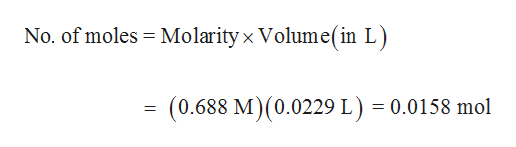# How many grams of solid potassium hydroxide are needed to exactly neutralize 22.9 mL of a 0.688 M hydrobromic acid solution ? Assume that the volume remains constant.

Question
1 views

How many grams of solid potassium hydroxide are needed to exactly neutralize 22.9 mL of a 0.688 M hydrobromic acid solution ? Assume that the volume remains constant.

check_circle

Step 1

Number of moles of hydrobromi...help_outlineImage TranscriptioncloseNo. of moles Molarity x Volume(in L) (0.688 M) (0.0229 L) = 0.0158 mol fullscreen

### Want to see the full answer?

See Solution

#### Want to see this answer and more?

Solutions are written by subject experts who are available 24/7. Questions are typically answered within 1 hour.*

See Solution
*Response times may vary by subject and question.
Tagged in

### General Chemistry